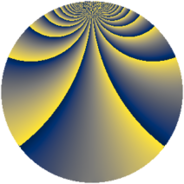# Properties

 Label 1045.2.buLevel $1045$ Weight $2$ Character orbit 1045.bu Rep. character $\chi_{1045}(49,\cdot)$ Character field $\Q(\zeta_{30})$ Dimension $928$ Sturm bound $240$

# Related objects

## Defining parameters

 Level: $$N$$ $$=$$ $$1045 = 5 \cdot 11 \cdot 19$$ Weight: $$k$$ $$=$$ $$2$$ Character orbit: $$[\chi]$$ $$=$$ 1045.bu (of order $$30$$ and degree $$8$$) Character conductor: $$\operatorname{cond}(\chi)$$ $$=$$ $$1045$$ Character field: $$\Q(\zeta_{30})$$ Sturm bound: $$240$$

## Dimensions

The following table gives the dimensions of various subspaces of $$M_{2}(1045, [\chi])$$.

Total New Old
Modular forms 992 992 0
Cusp forms 928 928 0
Eisenstein series 64 64 0

## Trace form

 $$928 q - 118 q^{4} - 5 q^{5} + 10 q^{6} - 114 q^{9} + O(q^{10})$$ $$928 q - 118 q^{4} - 5 q^{5} + 10 q^{6} - 114 q^{9} - 40 q^{11} - 8 q^{14} + 5 q^{15} + 90 q^{16} - 16 q^{19} - 116 q^{20} - 92 q^{21} - 22 q^{24} - 11 q^{25} - 20 q^{26} - 30 q^{29} - 94 q^{30} - 24 q^{31} - 27 q^{35} + 92 q^{36} + 38 q^{40} + 8 q^{41} + 14 q^{44} - 140 q^{45} - 152 q^{46} + 64 q^{49} + 22 q^{50} + 74 q^{51} - 76 q^{54} - 25 q^{55} - 216 q^{56} + 74 q^{59} + 22 q^{60} - 28 q^{61} - 24 q^{64} + 8 q^{65} + 76 q^{66} - 84 q^{69} + 2 q^{70} + 2 q^{71} + 14 q^{74} + 32 q^{75} + 84 q^{76} - 46 q^{79} + 83 q^{80} + 130 q^{81} + 184 q^{84} - 6 q^{85} + 22 q^{86} - 28 q^{89} - 7 q^{90} - 20 q^{91} + 56 q^{94} + 54 q^{95} + 4 q^{96} + 54 q^{99} + O(q^{100})$$

## Decomposition of $$S_{2}^{\mathrm{new}}(1045, [\chi])$$ into newform subspaces

The newforms in this space have not yet been added to the LMFDB.